# Create Beautiful Fractal Art

Fractals are proof that math can be visually beautiful. What are they exactly? A definition on Wikipedia states, “A fractal is a mathematical set that exhibits a repeating pattern displayed at every scale.” The best way to explain it is showing one of the oldest and simplest fractals – the Sierpinski triangle. It is created using an infinite process starting from an equilateral triangle. The triangle is split into four parts by connecting edge midpoints with straight lines. Then the middle part is removed. This leaves three smaller equilateral triangles. Applying the same step to each of them, one obtains nine even smaller triangles. Since the process is infinite, one sees the same repeating pattern no matter how far you zoom in.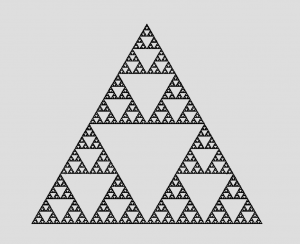Visually more attractive examples of fractals are the Mandelbrot and Julia sets. Here is a Mandelbrot: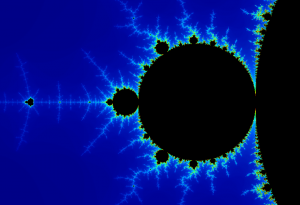The Julia set offers greater flexibility and more variations. All the images below are examples of the Julia sets created with the help of NCLab’s Fractal Explorer app, using different parameter values.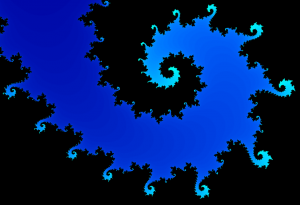This one is named “Eye See You”: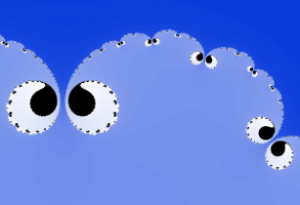This is the Dragon: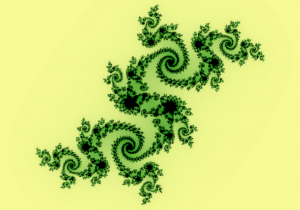The Sea Breeze: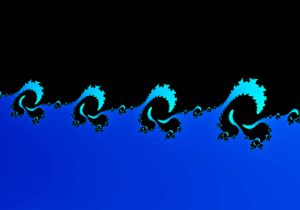And finally the Lizard: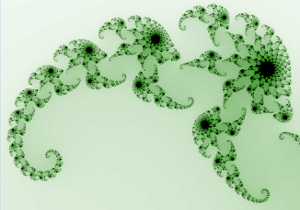Both Mandlebrot and Julia fractals can be explored using the Fractal Explorer app in NCLab’s Creative Suite – Math section. The Fractal Explorer allows students to zoom to infinite depths, discovering that exactly same shapes indeed repeat themselves on smaller and smaller scales. If you are interested in learning some of the math behind fractals, check out the Fractal Explorer Tutorial!  If you create a pretty design, we will be happy to add your art into the Fractal Art Gallery!